# Chapter 7 PRODUCTION FUNCTIONS 1 Production Function The

• Slides: 40Chapter 7 PRODUCTION FUNCTIONS 1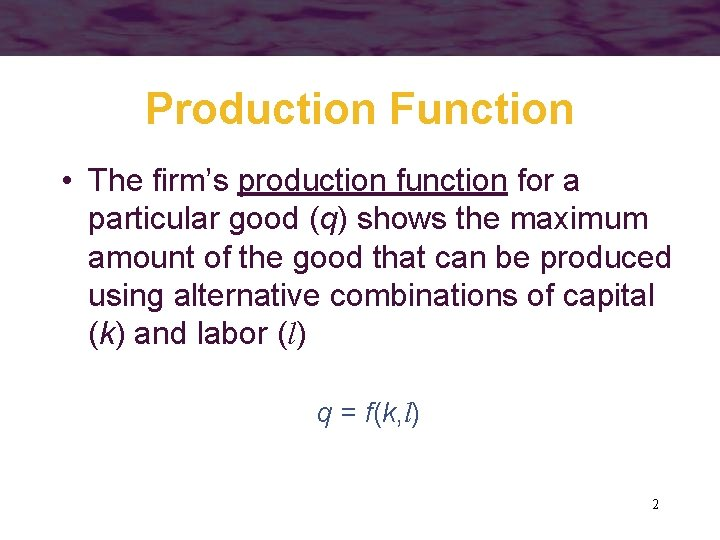Production Function • The firm’s production function for a particular good (q) shows the maximum amount of the good that can be produced using alternative combinations of capital (k) and labor (l) q = f(k, l) 2Marginal Physical Product • To study variation in a single input, we define marginal physical product as the additional output that can be produced by employing one more unit of that input while holding other inputs constant 3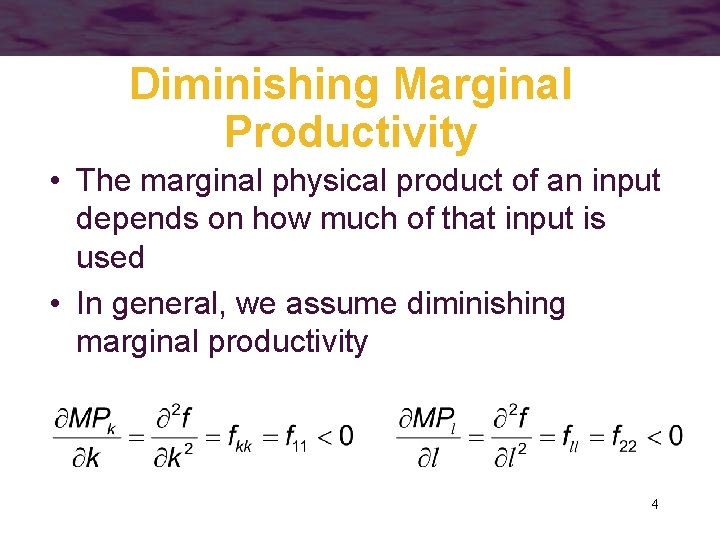Diminishing Marginal Productivity • The marginal physical product of an input depends on how much of that input is used • In general, we assume diminishing marginal productivity 4Diminishing Marginal Productivity • Because of diminishing marginal productivity, 19 th century economist Thomas Malthus worried about the effect of population growth on labor productivity • But changes in the marginal productivity of labor over time also depend on changes in other inputs such as capital – we need to consider flk which is often > 0 5Average Physical Product • Labor productivity is often measured by average productivity • Note that APl also depends on the amount of capital employed 6A Two-Input Production Function • Suppose the production function for flyswatters can be represented by q = f(k, l) = 600 k 2 l 2 - k 3 l 3 • To construct MPl and APl, we must assume a value for k – let k = 10 • The production function becomes q = 60, 000 l 2 - 1000 l 3 7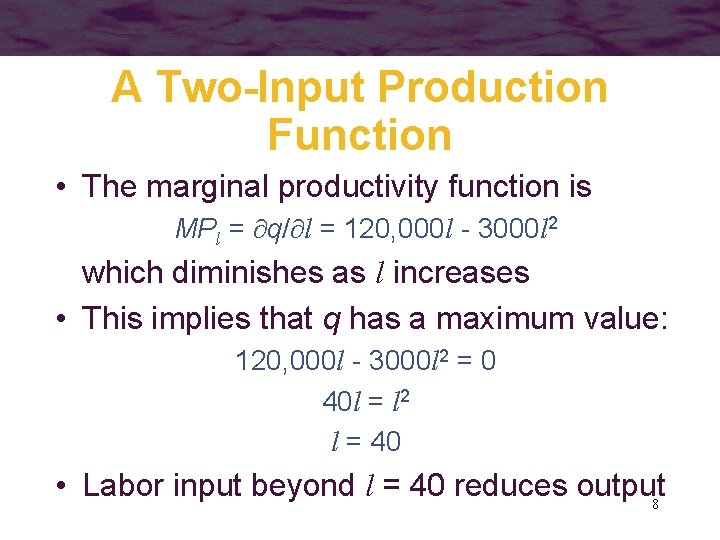A Two-Input Production Function • The marginal productivity function is MPl = q/ l = 120, 000 l - 3000 l 2 which diminishes as l increases • This implies that q has a maximum value: 120, 000 l - 3000 l 2 = 0 40 l = l 2 l = 40 • Labor input beyond l = 40 reduces output 8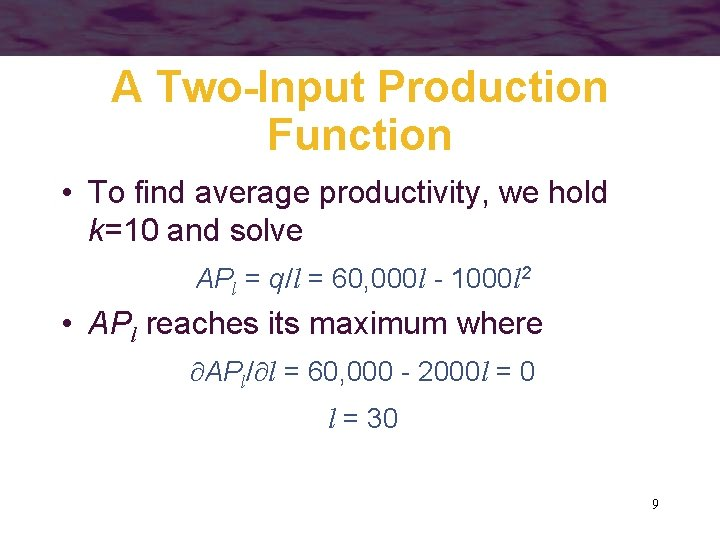A Two-Input Production Function • To find average productivity, we hold k=10 and solve APl = q/l = 60, 000 l - 1000 l 2 • APl reaches its maximum where APl/ l = 60, 000 - 2000 l = 0 l = 30 9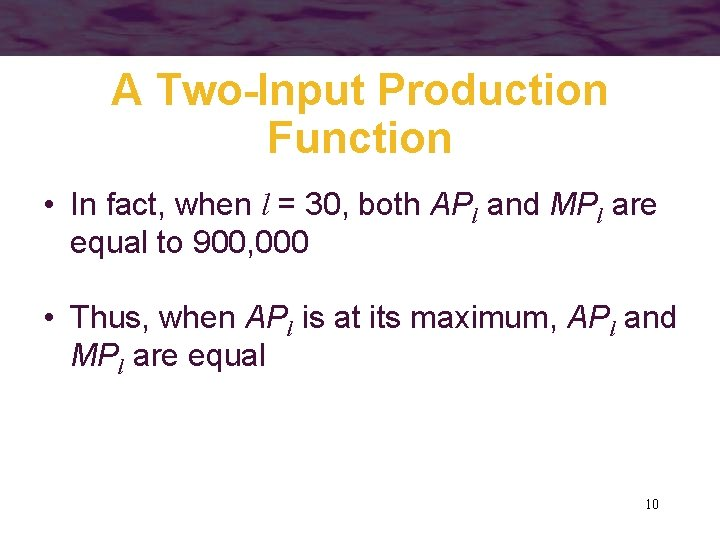A Two-Input Production Function • In fact, when l = 30, both APl and MPl are equal to 900, 000 • Thus, when APl is at its maximum, APl and MPl are equal 10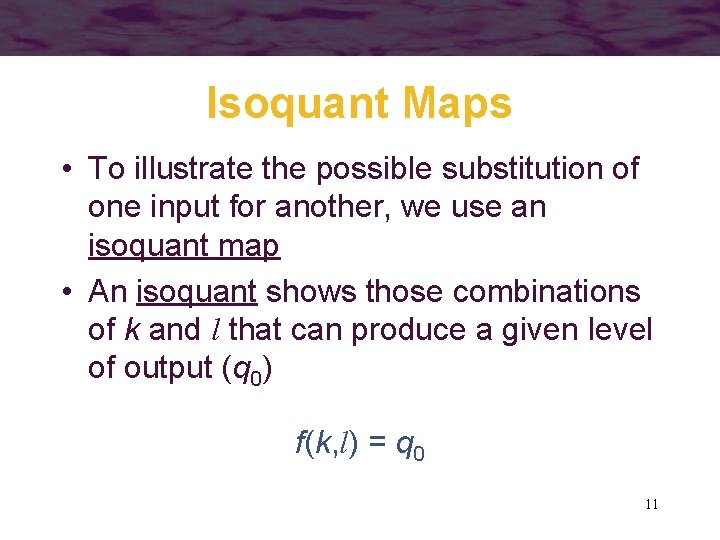Isoquant Maps • To illustrate the possible substitution of one input for another, we use an isoquant map • An isoquant shows those combinations of k and l that can produce a given level of output (q 0) f(k, l) = q 0 11Isoquant Map • Each isoquant represents a different level of output – output rises as we move northeast k period q = 30 q = 20 l period 12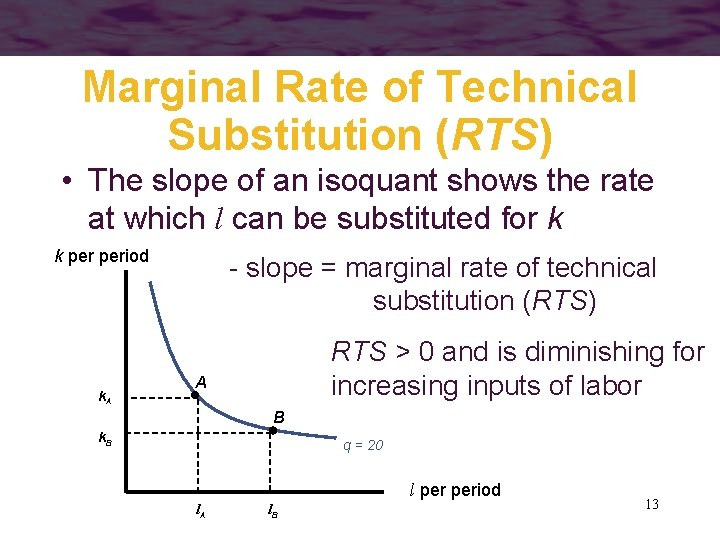Marginal Rate of Technical Substitution (RTS) • The slope of an isoquant shows the rate at which l can be substituted for k k period k. A - slope = marginal rate of technical substitution (RTS) RTS > 0 and is diminishing for increasing inputs of labor A B k. B q = 20 l period l. A l. B 13Marginal Rate of Technical Substitution (RTS) • The marginal rate of technical substitution (RTS) shows the rate at which labor can be substituted for capital while holding output constant along an isoquant 14RTS and Marginal Productivities • Take the total differential of the production function: • Along an isoquant dq = 0, so 15RTS and Marginal Productivities • Because MPl and MPk will both be nonnegative, RTS will be positive (or zero) • However, it is generally not possible to derive a diminishing RTS from the assumption of diminishing marginal productivity alone 16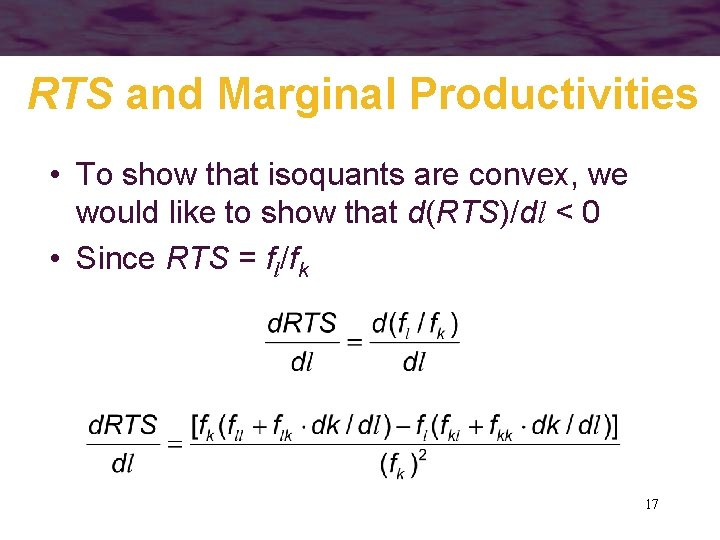RTS and Marginal Productivities • To show that isoquants are convex, we would like to show that d(RTS)/dl < 0 • Since RTS = fl/fk 17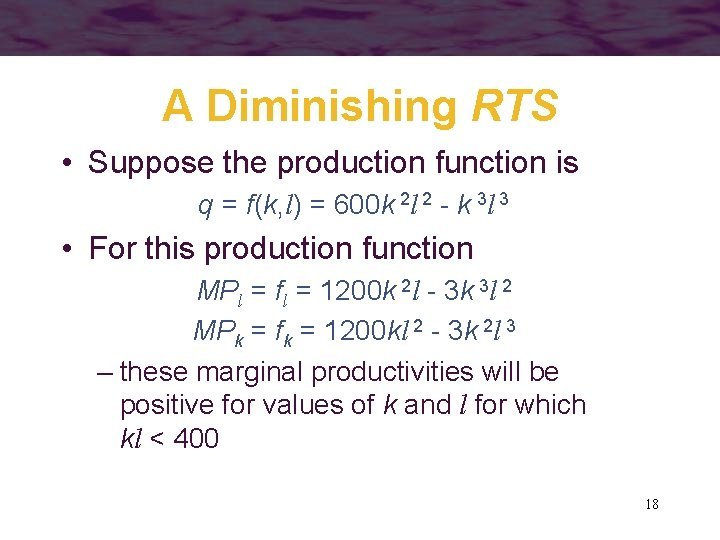A Diminishing RTS • Suppose the production function is q = f(k, l) = 600 k 2 l 2 - k 3 l 3 • For this production function MPl = fl = 1200 k 2 l - 3 k 3 l 2 MPk = fk = 1200 kl 2 - 3 k 2 l 3 – these marginal productivities will be positive for values of k and l for which kl < 400 18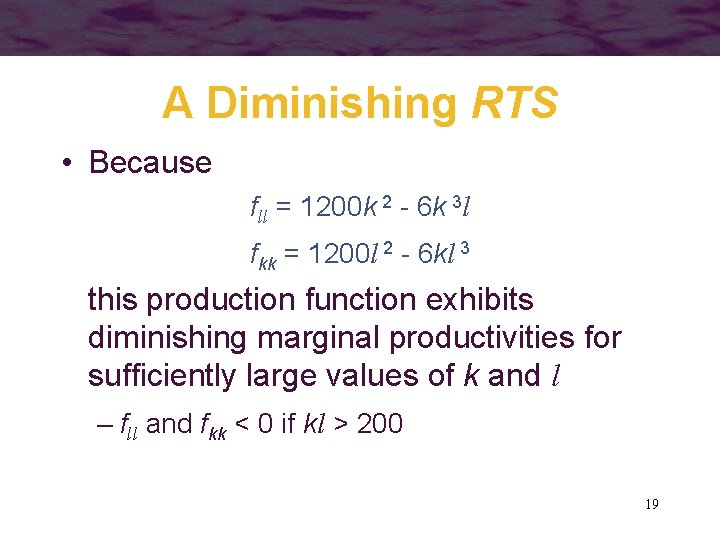A Diminishing RTS • Because fll = 1200 k 2 - 6 k 3 l fkk = 1200 l 2 - 6 kl 3 this production function exhibits diminishing marginal productivities for sufficiently large values of k and l – fll and fkk < 0 if kl > 200 19A Diminishing RTS • Cross differentiation of either of the marginal productivity functions yields fkl = flk = 2400 kl - 9 k 2 l 2 which is positive only for kl < 266 20A Diminishing RTS • Thus, for this production function, RTS is diminishing throughout the range of k and l where marginal productivities are positive – for higher values of k and l, the diminishing marginal productivities are sufficient to overcome the influence of a negative value for fkl to ensure convexity of the isoquants 21Returns to Scale • How does output respond to increases in all inputs together? – suppose that all inputs are doubled, would output double? • Returns to scale have been of interest to economists since the days of Adam Smith 22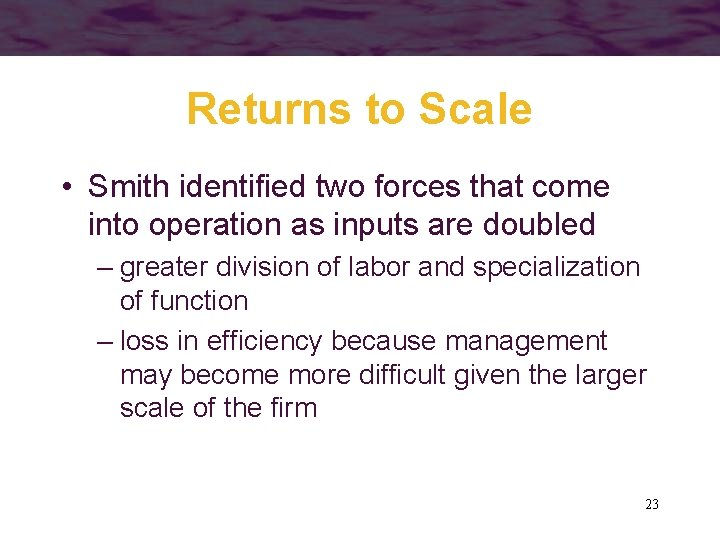Returns to Scale • Smith identified two forces that come into operation as inputs are doubled – greater division of labor and specialization of function – loss in efficiency because management may become more difficult given the larger scale of the firm 23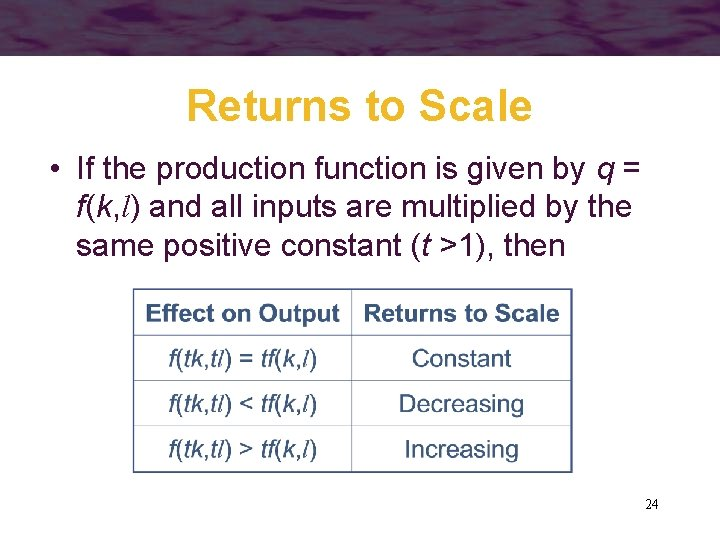Returns to Scale • If the production function is given by q = f(k, l) and all inputs are multiplied by the same positive constant (t >1), then 24Returns to Scale • It is possible for a production function to exhibit constant returns to scale for some levels of input usage and increasing or decreasing returns for other levels – economists refer to the degree of returns to scale with the implicit notion that only a fairly narrow range of variation in input usage and the related level of output is being considered 25Constant Returns to Scale • Constant returns-to-scale production functions are homogeneous of degree one in inputs f(tk, tl) = t 1 f(k, l) = tq • This implies that the marginal productivity functions are homogeneous of degree zero – if a function is homogeneous of degree k, its derivatives are homogeneous of degree 26 k-1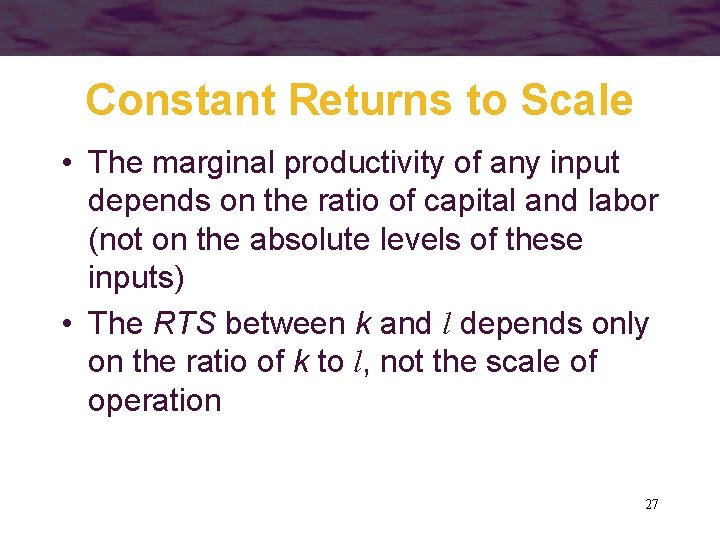Constant Returns to Scale • The marginal productivity of any input depends on the ratio of capital and labor (not on the absolute levels of these inputs) • The RTS between k and l depends only on the ratio of k to l, not the scale of operation 27Constant Returns to Scale • The production function will be homothetic • Geometrically, all of the isoquants are radial expansions of one another 28Constant Returns to Scale • Along a ray from the origin (constant k/l), the RTS will be the same on all isoquants k period The isoquants are equally spaced as output expands q=3 q=2 q=1 l period 29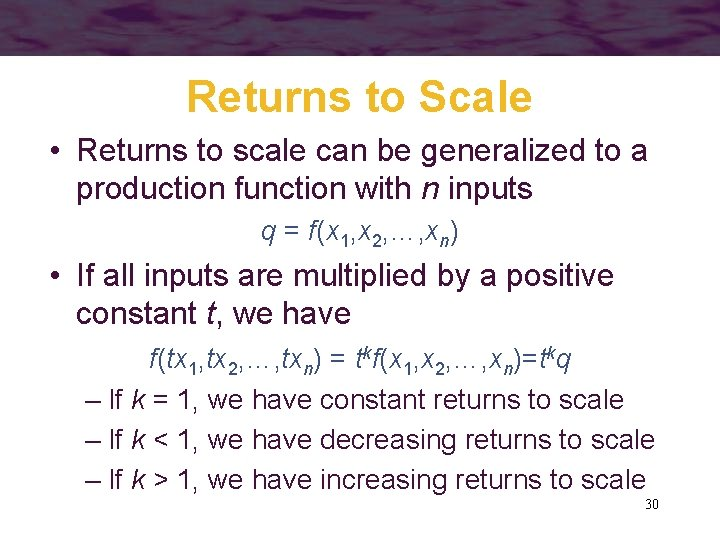Returns to Scale • Returns to scale can be generalized to a production function with n inputs q = f(x 1, x 2, …, xn) • If all inputs are multiplied by a positive constant t, we have f(tx 1, tx 2, …, txn) = tkf(x 1, x 2, …, xn)=tkq – If k = 1, we have constant returns to scale – If k < 1, we have decreasing returns to scale – If k > 1, we have increasing returns to scale 30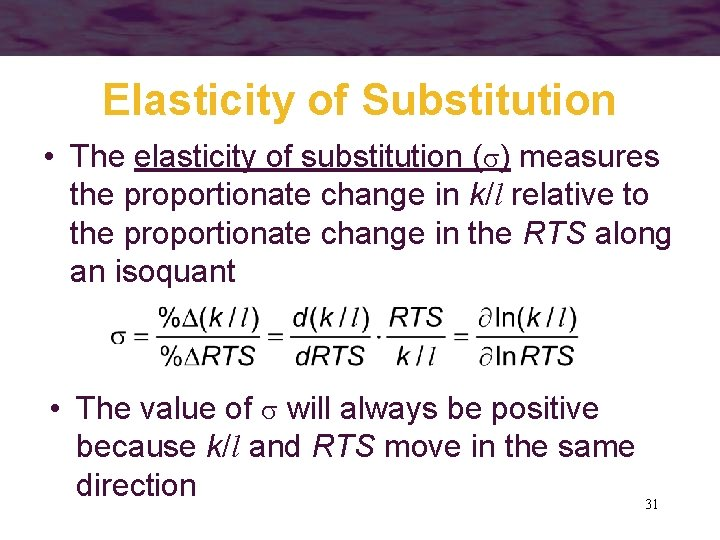Elasticity of Substitution • The elasticity of substitution ( ) measures the proportionate change in k/l relative to the proportionate change in the RTS along an isoquant • The value of will always be positive because k/l and RTS move in the same direction 31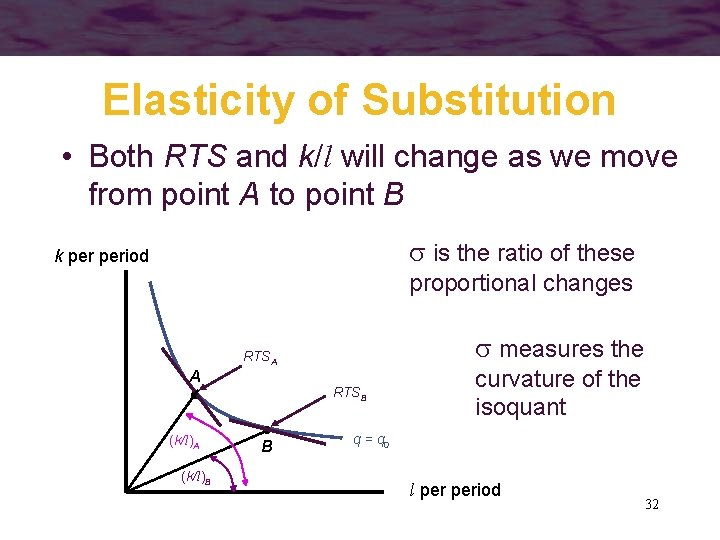Elasticity of Substitution • Both RTS and k/l will change as we move from point A to point B is the ratio of these k period proportional changes measures the RTSA A (k/l)B RTSB B curvature of the isoquant q = q 0 l period 32Elasticity of Substitution • If is high, the RTS will not change much relative to k/l – the isoquant will be relatively flat • If is low, the RTS will change by a substantial amount as k/l changes – the isoquant will be sharply curved • It is possible for to change along an isoquant or as the scale of production changes 33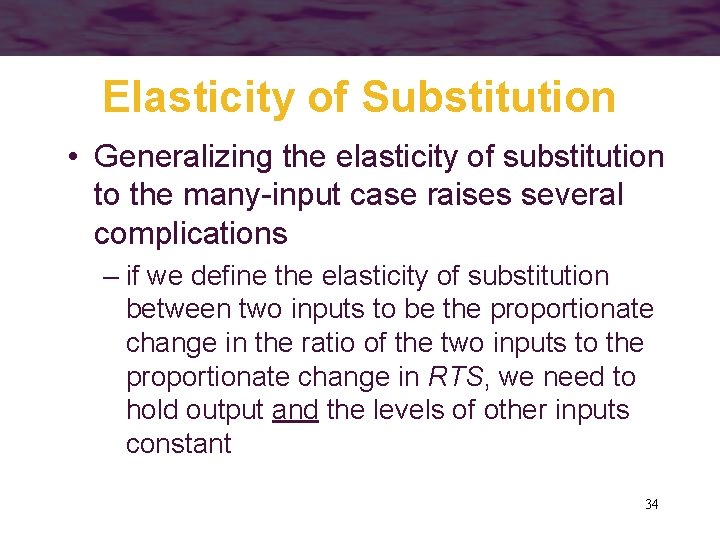Elasticity of Substitution • Generalizing the elasticity of substitution to the many-input case raises several complications – if we define the elasticity of substitution between two inputs to be the proportionate change in the ratio of the two inputs to the proportionate change in RTS, we need to hold output and the levels of other inputs constant 34The Linear Production Function • Suppose that the production function is q = f(k, l) = ak + bl • This production function exhibits constant returns to scale f(tk, tl) = atk + btl = t(ak + bl) = tf(k, l) • All isoquants are straight lines – RTS is constant – = 35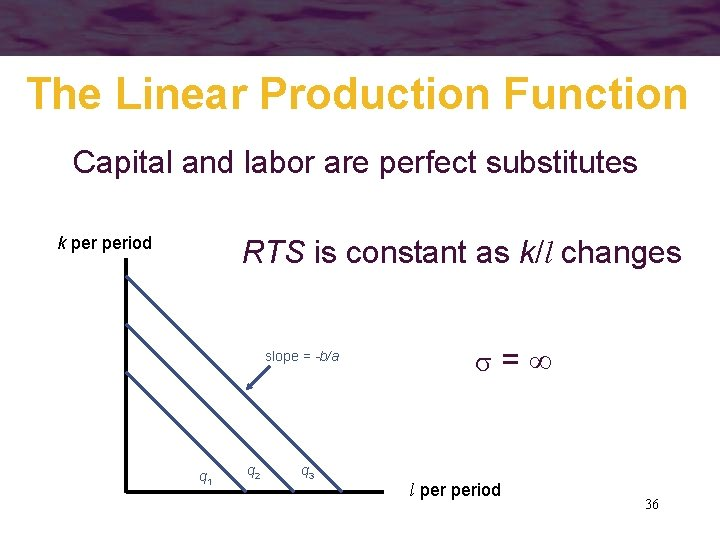The Linear Production Function Capital and labor are perfect substitutes k period RTS is constant as k/l changes slope = -b/a q 1 q 2 q 3 = l period 36Fixed Proportions • Suppose that the production function is q = min (ak, bl) a, b > 0 • Capital and labor must always be used in a fixed ratio – the firm will always operate along a ray where k/l is constant • Because k/l is constant, = 0 37Fixed Proportions No substitution between labor and capital is possible k/l is fixed at b/a k period =0 q 3/a q 2 q 1 q 3/b l period 38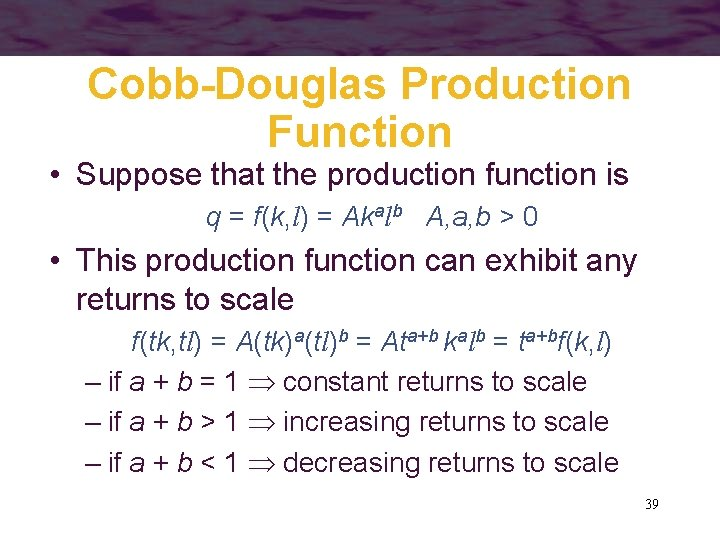Cobb-Douglas Production Function • Suppose that the production function is q = f(k, l) = Akalb A, a, b > 0 • This production function can exhibit any returns to scale f(tk, tl) = A(tk)a(tl)b = Ata+b kalb = ta+bf(k, l) – if a + b = 1 constant returns to scale – if a + b > 1 increasing returns to scale – if a + b < 1 decreasing returns to scale 39Cobb-Douglas Production Function • The Cobb-Douglas production function is linear in logarithms ln q = ln A + a ln k + b ln l – a is the elasticity of output with respect to k – b is the elasticity of output with respect to l 40